# Lecture-2.pptx

30 May 2023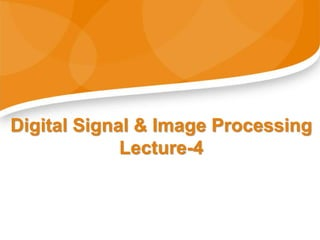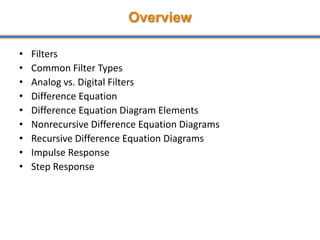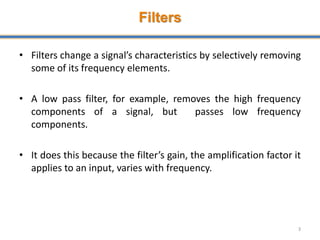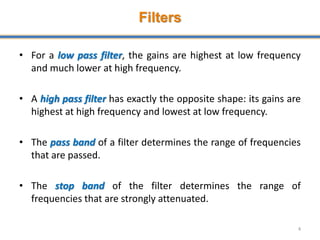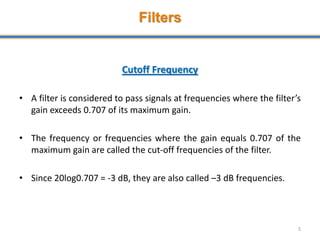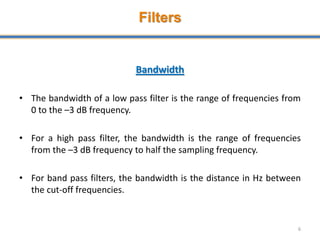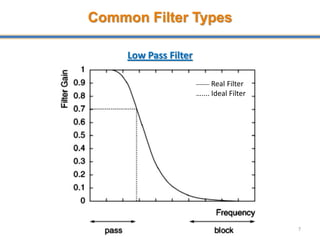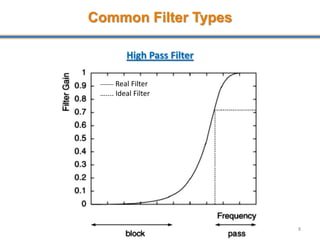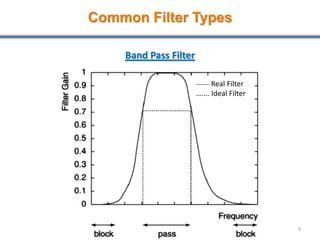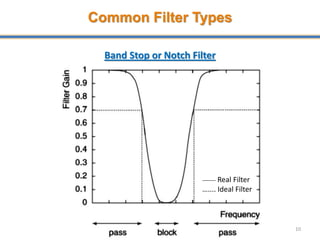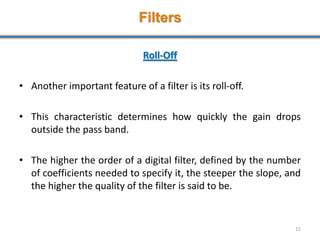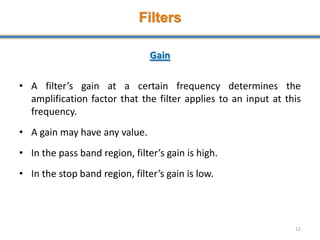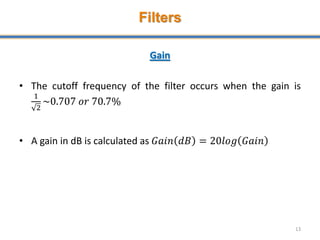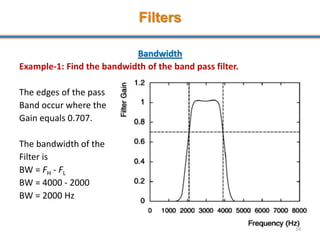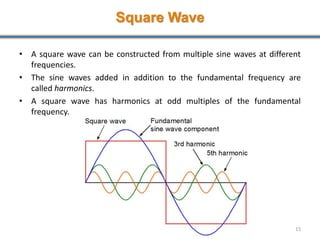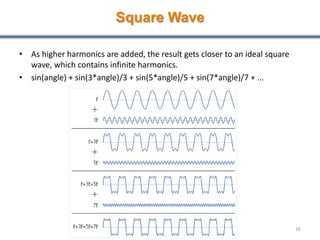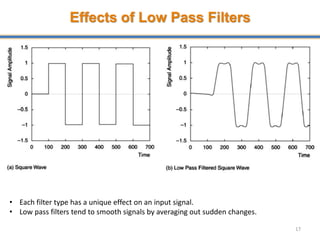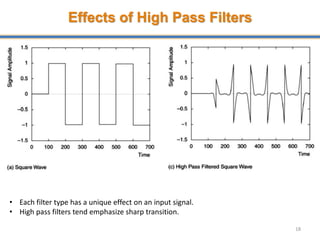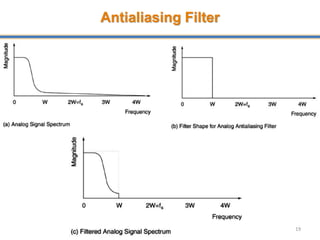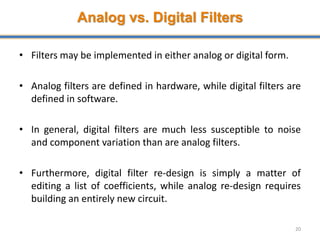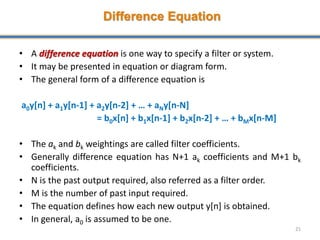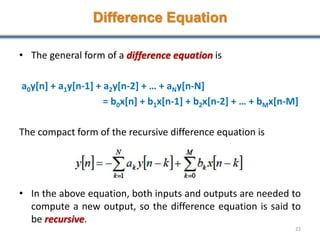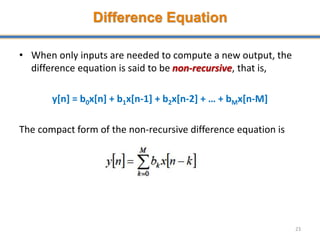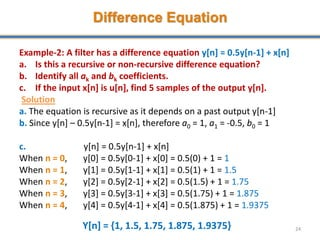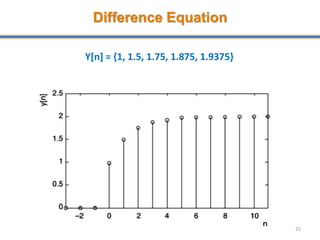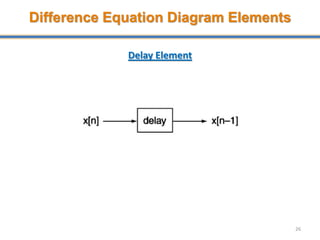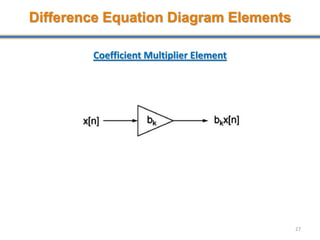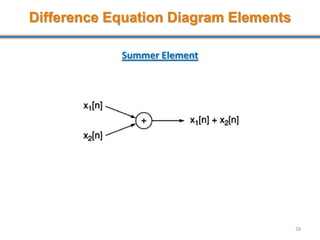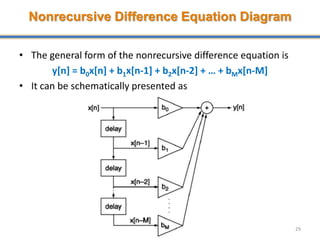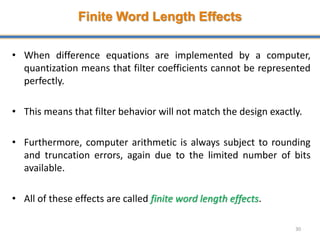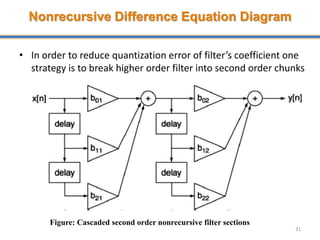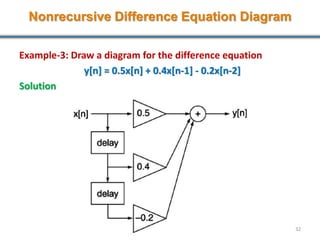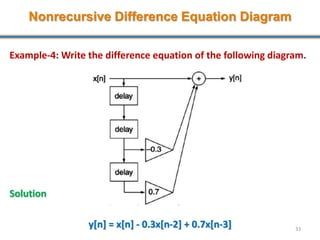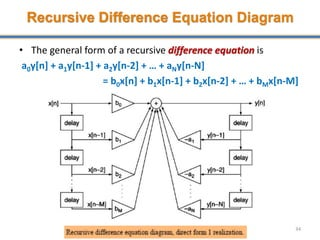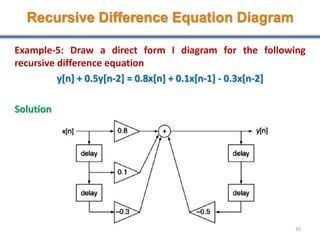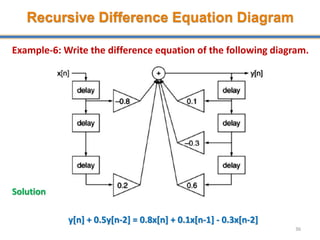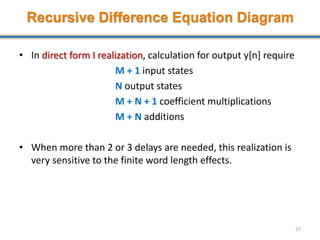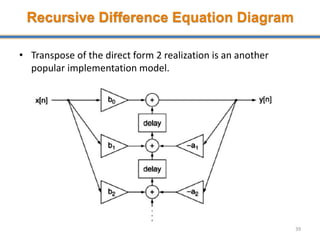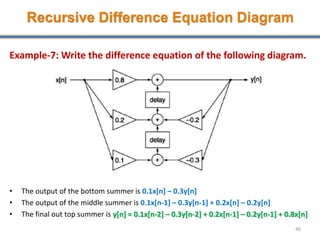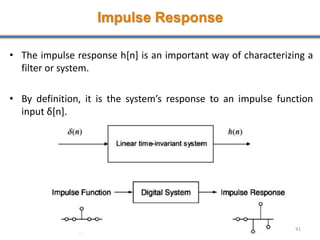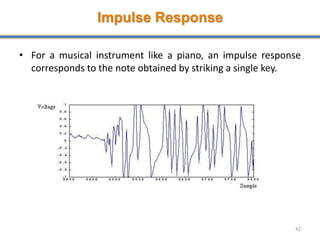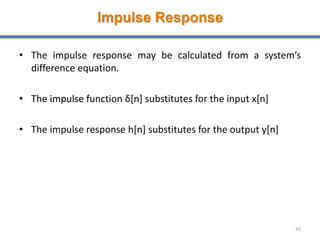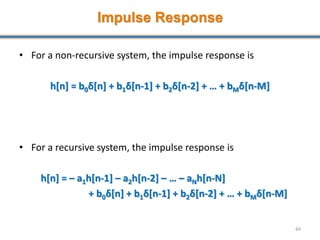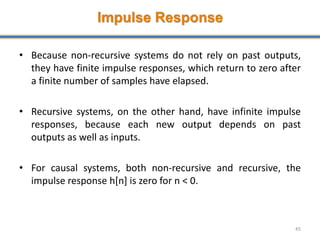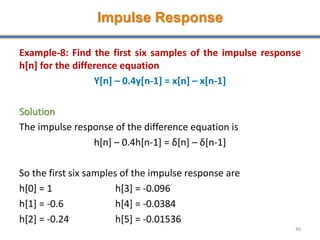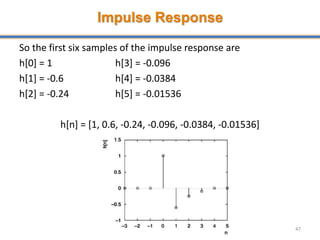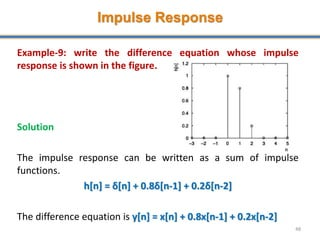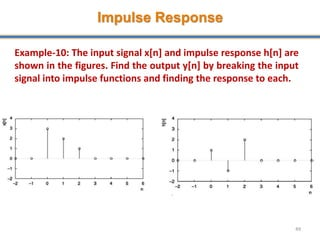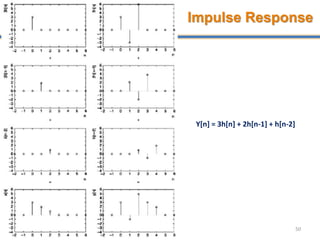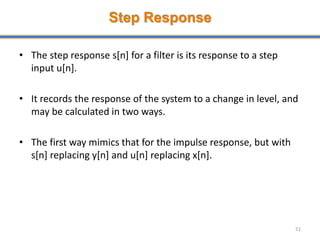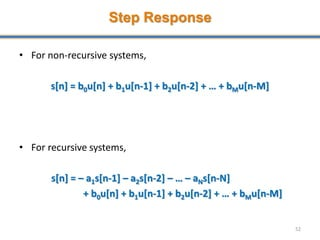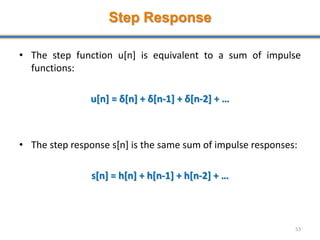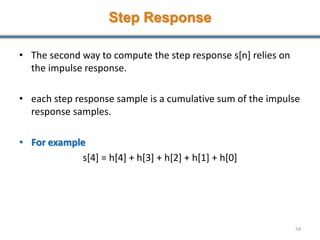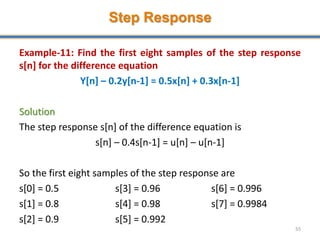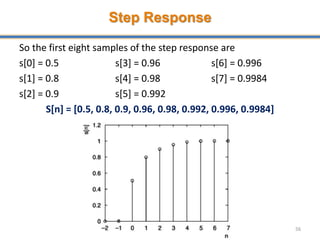1 sur 56

### Lecture-2.pptx

• 1. Digital Signal & Image Processing Lecture-4
• 2. Overview • Filters • Common Filter Types • Analog vs. Digital Filters • Difference Equation • Difference Equation Diagram Elements • Nonrecursive Difference Equation Diagrams • Recursive Difference Equation Diagrams • Impulse Response • Step Response
• 3. Filters • Filters change a signal’s characteristics by selectively removing some of its frequency elements. • A low pass filter, for example, removes the high frequency components of a signal, but passes low frequency components. • It does this because the filter’s gain, the amplification factor it applies to an input, varies with frequency. 3
• 4. Filters • For a low pass filter, the gains are highest at low frequency and much lower at high frequency. • A high pass filter has exactly the opposite shape: its gains are highest at high frequency and lowest at low frequency. • The pass band of a filter determines the range of frequencies that are passed. • The stop band of the filter determines the range of frequencies that are strongly attenuated. 4
• 5. Filters Cutoff Frequency • A filter is considered to pass signals at frequencies where the filter’s gain exceeds 0.707 of its maximum gain. • The frequency or frequencies where the gain equals 0.707 of the maximum gain are called the cut-off frequencies of the filter. • Since 20log0.707 = -3 dB, they are also called –3 dB frequencies. 5
• 6. Filters Bandwidth • The bandwidth of a low pass filter is the range of frequencies from 0 to the –3 dB frequency. • For a high pass filter, the bandwidth is the range of frequencies from the –3 dB frequency to half the sampling frequency. • For band pass filters, the bandwidth is the distance in Hz between the cut-off frequencies. 6
• 7. Common Filter Types Low Pass Filter 7 _____ Real Filter ….... Ideal Filter
• 8. Common Filter Types High Pass Filter 8 _____ Real Filter ….... Ideal Filter
• 9. Common Filter Types Band Pass Filter 9 _____ Real Filter ….... Ideal Filter
• 10. Common Filter Types Band Stop or Notch Filter 10 _____ Real Filter ….... Ideal Filter
• 11. Filters Roll-Off • Another important feature of a filter is its roll-off. • This characteristic determines how quickly the gain drops outside the pass band. • The higher the order of a digital filter, defined by the number of coefficients needed to specify it, the steeper the slope, and the higher the quality of the filter is said to be. 11
• 12. Filters Gain • A filter’s gain at a certain frequency determines the amplification factor that the filter applies to an input at this frequency. • A gain may have any value. • In the pass band region, filter’s gain is high. • In the stop band region, filter’s gain is low. 12
• 13. Filters Gain • The cutoff frequency of the filter occurs when the gain is 1 2 ~0.707 𝑜𝑟 70.7% • A gain in dB is calculated as 𝐺𝑎𝑖𝑛 𝑑𝐵 = 20𝑙𝑜𝑔 𝐺𝑎𝑖𝑛 13
• 14. Filters Bandwidth Example-1: Find the bandwidth of the band pass filter. The edges of the pass Band occur where the Gain equals 0.707. The bandwidth of the Filter is BW = FH - FL BW = 4000 - 2000 BW = 2000 Hz 14
• 15. Square Wave • A square wave can be constructed from multiple sine waves at different frequencies. • The sine waves added in addition to the fundamental frequency are called harmonics. • A square wave has harmonics at odd multiples of the fundamental frequency. 15
• 16. Square Wave • As higher harmonics are added, the result gets closer to an ideal square wave, which contains infinite harmonics. • sin(angle) + sin(3*angle)/3 + sin(5*angle)/5 + sin(7*angle)/7 + ... 16
• 17. Effects of Low Pass Filters 17 • Each filter type has a unique effect on an input signal. • Low pass filters tend to smooth signals by averaging out sudden changes.
• 18. Effects of High Pass Filters 18 • Each filter type has a unique effect on an input signal. • High pass filters tend emphasize sharp transition.
• 19. Antialiasing Filter 19
• 20. Analog vs. Digital Filters • Filters may be implemented in either analog or digital form. • Analog filters are defined in hardware, while digital filters are defined in software. • In general, digital filters are much less susceptible to noise and component variation than are analog filters. • Furthermore, digital filter re-design is simply a matter of editing a list of coefficients, while analog re-design requires building an entirely new circuit. 20
• 21. Difference Equation • A difference equation is one way to specify a filter or system. • It may be presented in equation or diagram form. • The general form of a difference equation is a0y[n] + a1y[n-1] + a2y[n-2] + … + aNy[n-N] = b0x[n] + b1x[n-1] + b2x[n-2] + … + bMx[n-M] • The ak and bk weightings are called filter coefficients. • Generally difference equation has N+1 ak coefficients and M+1 bk coefficients. • N is the past output required, also referred as a filter order. • M is the number of past input required. • The equation defines how each new output y[n] is obtained. • In general, a0 is assumed to be one. 21
• 22. Difference Equation • The general form of a difference equation is a0y[n] + a1y[n-1] + a2y[n-2] + … + aNy[n-N] = b0x[n] + b1x[n-1] + b2x[n-2] + … + bMx[n-M] The compact form of the recursive difference equation is • In the above equation, both inputs and outputs are needed to compute a new output, so the difference equation is said to be recursive. 22
• 23. Difference Equation • When only inputs are needed to compute a new output, the difference equation is said to be non-recursive, that is, y[n] = b0x[n] + b1x[n-1] + b2x[n-2] + … + bMx[n-M] The compact form of the non-recursive difference equation is 23
• 24. Difference Equation Example-2: A filter has a difference equation y[n] = 0.5y[n-1] + x[n] a. Is this a recursive or non-recursive difference equation? b. Identify all ak and bk coefficients. c. If the input x[n] is u[n], find 5 samples of the output y[n]. Solution a. The equation is recursive as it depends on a past output y[n-1] b. Since y[n] – 0.5y[n-1] = x[n], therefore a0 = 1, a1 = -0.5, b0 = 1 c. y[n] = 0.5y[n-1] + x[n] When n = 0, y = 0.5y[0-1] + x = 0.5(0) + 1 = 1 When n = 1, y = 0.5y[1-1] + x = 0.5(1) + 1 = 1.5 When n = 2, y = 0.5y[2-1] + x = 0.5(1.5) + 1 = 1.75 When n = 3, y = 0.5y[3-1] + x = 0.5(1.75) + 1 = 1.875 When n = 4, y = 0.5y[4-1] + x = 0.5(1.875) + 1 = 1.9375 24 Y[n] = {1, 1.5, 1.75, 1.875, 1.9375}
• 25. Difference Equation 25 Y[n] = {1, 1.5, 1.75, 1.875, 1.9375}
• 26. Difference Equation Diagram Elements Delay Element 26
• 27. Difference Equation Diagram Elements Coefficient Multiplier Element 27
• 28. Difference Equation Diagram Elements Summer Element 28
• 29. Nonrecursive Difference Equation Diagram • The general form of the nonrecursive difference equation is y[n] = b0x[n] + b1x[n-1] + b2x[n-2] + … + bMx[n-M] • It can be schematically presented as 29
• 30. Finite Word Length Effects • When difference equations are implemented by a computer, quantization means that filter coefficients cannot be represented perfectly. • This means that filter behavior will not match the design exactly. • Furthermore, computer arithmetic is always subject to rounding and truncation errors, again due to the limited number of bits available. • All of these effects are called finite word length effects. 30
• 31. Nonrecursive Difference Equation Diagram • In order to reduce quantization error of filter’s coefficient one strategy is to break higher order filter into second order chunks 31 Figure: Cascaded second order nonrecursive filter sections
• 32. Nonrecursive Difference Equation Diagram Example-3: Draw a diagram for the difference equation y[n] = 0.5x[n] + 0.4x[n-1] - 0.2x[n-2] Solution 32
• 33. Nonrecursive Difference Equation Diagram Example-4: Write the difference equation of the following diagram. Solution y[n] = x[n] - 0.3x[n-2] + 0.7x[n-3] 33
• 34. Recursive Difference Equation Diagram • The general form of a recursive difference equation is a0y[n] + a1y[n-1] + a2y[n-2] + … + aNy[n-N] = b0x[n] + b1x[n-1] + b2x[n-2] + … + bMx[n-M] 34
• 35. Recursive Difference Equation Diagram Example-5: Draw a direct form I diagram for the following recursive difference equation y[n] + 0.5y[n-2] = 0.8x[n] + 0.1x[n-1] - 0.3x[n-2] Solution 35
• 36. Recursive Difference Equation Diagram Example-6: Write the difference equation of the following diagram. Solution y[n] + 0.5y[n-2] = 0.8x[n] + 0.1x[n-1] - 0.3x[n-2] 36
• 37. Recursive Difference Equation Diagram • In direct form I realization, calculation for output y[n] require M + 1 input states N output states M + N + 1 coefficient multiplications M + N additions • When more than 2 or 3 delays are needed, this realization is very sensitive to the finite word length effects. 37
• 38. Recursive Difference Equation Diagram • Recursive filters have an alternative representation, called direct form 2, that has certain advantages for implementation. • This representation is defined by the pair of equations w[n] = x[n] – a1w[n-1] – a2w[n-2] – … – aNw[n-N] y[n] = b0w[n] + b1w[n-1] + b2w[n-2] + … + bMw[n-M] 38
• 39. Recursive Difference Equation Diagram • Transpose of the direct form 2 realization is an another popular implementation model. 39
• 40. Recursive Difference Equation Diagram Example-7: Write the difference equation of the following diagram. • The output of the bottom summer is 0.1x[n] – 0.3y[n] • The output of the middle summer is 0.1x[n-1] – 0.3y[n-1] + 0.2x[n] – 0.2y[n] • The final out top summer is y[n] = 0.1x[n-2] – 0.3y[n-2] + 0.2x[n-1] – 0.2y[n-1] + 0.8x[n] 40
• 41. Impulse Response • The impulse response h[n] is an important way of characterizing a filter or system. • By definition, it is the system’s response to an impulse function input δ[n]. 41
• 42. Impulse Response • For a musical instrument like a piano, an impulse response corresponds to the note obtained by striking a single key. 42
• 43. Impulse Response • The impulse response may be calculated from a system’s difference equation. • The impulse function δ[n] substitutes for the input x[n] • The impulse response h[n] substitutes for the output y[n] 43
• 44. Impulse Response • For a non-recursive system, the impulse response is h[n] = b0δ[n] + b1δ[n-1] + b2δ[n-2] + … + bMδ[n-M] • For a recursive system, the impulse response is h[n] = – a1h[n-1] – a2h[n-2] – … – aNh[n-N] + b0δ[n] + b1δ[n-1] + b2δ[n-2] + … + bMδ[n-M] 44
• 45. Impulse Response • Because non-recursive systems do not rely on past outputs, they have finite impulse responses, which return to zero after a finite number of samples have elapsed. • Recursive systems, on the other hand, have infinite impulse responses, because each new output depends on past outputs as well as inputs. • For causal systems, both non-recursive and recursive, the impulse response h[n] is zero for n < 0. 45
• 46. Impulse Response Example-8: Find the first six samples of the impulse response h[n] for the difference equation Y[n] – 0.4y[n-1] = x[n] – x[n-1] Solution The impulse response of the difference equation is h[n] – 0.4h[n-1] = δ[n] – δ[n-1] So the first six samples of the impulse response are h = 1 h = -0.096 h = -0.6 h = -0.0384 h = -0.24 h = -0.01536 46
• 47. Impulse Response So the first six samples of the impulse response are h = 1 h = -0.096 h = -0.6 h = -0.0384 h = -0.24 h = -0.01536 h[n] = [1, 0.6, -0.24, -0.096, -0.0384, -0.01536] 47
• 48. Impulse Response Example-9: write the difference equation whose impulse response is shown in the figure. Solution The impulse response can be written as a sum of impulse functions. h[n] = δ[n] + 0.8δ[n-1] + 0.2δ[n-2] The difference equation is y[n] = x[n] + 0.8x[n-1] + 0.2x[n-2] 48
• 49. Impulse Response Example-10: The input signal x[n] and impulse response h[n] are shown in the figures. Find the output y[n] by breaking the input signal into impulse functions and finding the response to each. 49
• 50. Impulse Response 50 Y[n] = 3h[n] + 2h[n-1] + h[n-2]
• 51. Step Response • The step response s[n] for a filter is its response to a step input u[n]. • It records the response of the system to a change in level, and may be calculated in two ways. • The first way mimics that for the impulse response, but with s[n] replacing y[n] and u[n] replacing x[n]. 51
• 52. Step Response • For non-recursive systems, s[n] = b0u[n] + b1u[n-1] + b2u[n-2] + … + bMu[n-M] • For recursive systems, s[n] = – a1s[n-1] – a2s[n-2] – … – aNs[n-N] + b0u[n] + b1u[n-1] + b2u[n-2] + … + bMu[n-M] 52
• 53. Step Response • The step function u[n] is equivalent to a sum of impulse functions: u[n] = δ[n] + δ[n-1] + δ[n-2] + … • The step response s[n] is the same sum of impulse responses: s[n] = h[n] + h[n-1] + h[n-2] + … 53
• 54. Step Response • The second way to compute the step response s[n] relies on the impulse response. • each step response sample is a cumulative sum of the impulse response samples. • For example s = h + h + h + h + h 54
• 55. Step Response Example-11: Find the first eight samples of the step response s[n] for the difference equation Y[n] – 0.2y[n-1] = 0.5x[n] + 0.3x[n-1] Solution The step response s[n] of the difference equation is s[n] – 0.4s[n-1] = u[n] – u[n-1] So the first eight samples of the step response are s = 0.5 s = 0.96 s = 0.996 s = 0.8 s = 0.98 s = 0.9984 s = 0.9 s = 0.992 55
• 56. Step Response So the first eight samples of the step response are s = 0.5 s = 0.96 s = 0.996 s = 0.8 s = 0.98 s = 0.9984 s = 0.9 s = 0.992 S[n] = [0.5, 0.8, 0.9, 0.96, 0.98, 0.992, 0.996, 0.9984] 56